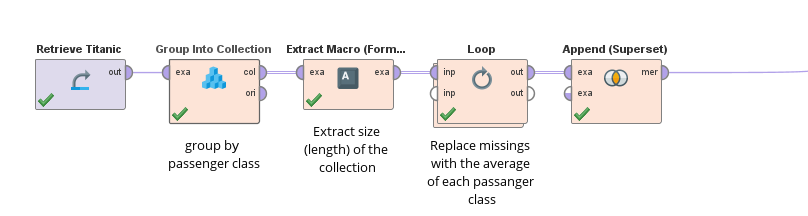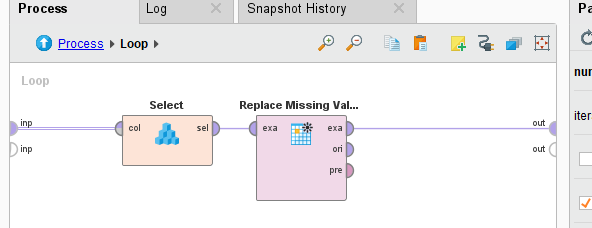# processing across batches

Moderator, RapidMiner Certified Analyst, RapidMiner Certified Expert, Member Posts: 1,635Unicorn
I am wondering what is the easiest way of doing a bunch of data ETL preprocessing on a large Exampleset in batches and keeping everything in RapidMiner.
There is an operator called Loop Batches, which in theory looks ideal, except it doesn't return the results from the batches and also doesn't include any batch macro to allow storage of intermediate results!  It looks like it is only suitable for use with databases, so it actually has very limited value.  Perhaps that operator could be expanded in the future to be more useful.
In the meantime, if you had 10k total records and wanted to divide them into 10 batches of 1000 records each, do a bunch of preprocessing, and then unify the resulting data at the end, what is the most efficient way to do this in RapidMiner?
Right now it looks like creating a batch attribute manually and then using the generic Loop operator, using Store on each intermediate batch/iteration result, and then using Append at the end.  But there might be some other creative way of using loops and macros that I am missing?

Brian T.
Lindon Ventures
Data Science Consulting from Certified RapidMiner Experts

• Administrator, Moderator, Employee, RapidMiner Certified Analyst, RapidMiner Certified Expert, University Professor Posts: 3,453RM Data Scientist
Solution Accepted

usually I use the Group Into Collection operator for it. You can simply create a collection with one example set per batch. Than you can use Loop Collection to loop over it and do what ever transformation on it.

The Group Collection + Loop Collection combo allows you to have somewhat a GROUP BY statement for any subprocess.

Its worth of note, that this duplicates the data once, since the items in the collection are a copy of the original data.

Loop Collection is also a loop operator which does not run in parallel. To overcome this I have implemented the option to extract the collection size in Extract Macro Format. This way you can then use the normal loop operator like this:And inside the loop you then select the i-th element:Attached is the example as a full work flow.

Cheers,
Martin

<?xml version="1.0" encoding="UTF-8"?><process version="9.8.001">
<context>
<input/>
<output/>
<macros/>
</context>
<operator activated="true" class="process" compatibility="9.8.001" expanded="true" name="Process">
<parameter key="logverbosity" value="init"/>
<parameter key="random_seed" value="2001"/>
<parameter key="send_mail" value="never"/>
<parameter key="process_duration_for_mail" value="30"/>
<parameter key="encoding" value="SYSTEM"/>
<process expanded="true">
<operator activated="true" class="retrieve" compatibility="9.8.001" expanded="true" height="68" name="Retrieve Titanic" width="90" x="112" y="136">
<parameter key="repository_entry" value="//Samples/data/Titanic"/>
</operator>
<operator activated="true" class="operator_toolbox:group_into_collection" compatibility="2.9.000-SNAPSHOT" expanded="true" height="82" name="Group Into Collection" width="90" x="246" y="136">
<parameter key="group_by_attribute" value="Passenger Class"/>
<parameter key="group_by_attribute (numerical)" value=""/>
<parameter key="sorting_order" value="none"/>
<description align="center" color="transparent" colored="false" width="126">group by passenger class</description>
</operator>
<operator activated="true" class="operator_toolbox:extract_macro_enhanced" compatibility="2.9.000-SNAPSHOT" expanded="true" height="68" name="Extract Macro (Format)" width="90" x="380" y="136">
<parameter key="macro" value="size"/>
<parameter key="macro_type" value="collection_size"/>
<parameter key="statistics" value="average"/>
<parameter key="attribute_name" value=""/>
<parameter key="format_of_numericals" value="#.##"/>
<parameter key="date_format" value=""/>
<parameter key="time_zone" value="SYSTEM"/>
<parameter key="locale" value="English (United States)"/>
<description align="center" color="transparent" colored="false" width="126">Extract size (length) of the collection</description>
</operator>
<operator activated="true" class="concurrency:loop" compatibility="9.8.001" expanded="true" height="82" name="Loop" width="90" x="514" y="136">
<parameter key="number_of_iterations" value="%{size}"/>
<parameter key="iteration_macro" value="iteration"/>
<parameter key="reuse_results" value="false"/>
<parameter key="enable_parallel_execution" value="true"/>
<process expanded="true">
<operator activated="true" class="select" compatibility="9.8.001" expanded="true" height="68" name="Select" width="90" x="112" y="34">
<parameter key="index" value="%{iteration}"/>
<parameter key="unfold" value="false"/>
</operator>
<operator activated="true" class="replace_missing_values" compatibility="9.8.001" expanded="true" height="103" name="Replace Missing Values" width="90" x="246" y="34">
<parameter key="return_preprocessing_model" value="false"/>
<parameter key="create_view" value="false"/>
<parameter key="attribute_filter_type" value="all"/>
<parameter key="attribute" value=""/>
<parameter key="attributes" value=""/>
<parameter key="use_except_expression" value="false"/>
<parameter key="value_type" value="attribute_value"/>
<parameter key="use_value_type_exception" value="false"/>
<parameter key="except_value_type" value="time"/>
<parameter key="block_type" value="attribute_block"/>
<parameter key="use_block_type_exception" value="false"/>
<parameter key="except_block_type" value="value_matrix_row_start"/>
<parameter key="invert_selection" value="false"/>
<parameter key="include_special_attributes" value="false"/>
<parameter key="default" value="average"/>
<list key="columns"/>
</operator>
<connect from_port="input 1" to_op="Select" to_port="collection"/>
<connect from_op="Select" from_port="selected" to_op="Replace Missing Values" to_port="example set input"/>
<connect from_op="Replace Missing Values" from_port="example set output" to_port="output 1"/>
<portSpacing port="source_input 1" spacing="0"/>
<portSpacing port="source_input 2" spacing="0"/>
<portSpacing port="sink_output 1" spacing="0"/>
<portSpacing port="sink_output 2" spacing="0"/>
</process>
<description align="center" color="transparent" colored="false" width="126">Replace missings with the average of each passanger class</description>
</operator>
<operator activated="true" class="operator_toolbox:advanced_append" compatibility="2.9.000-SNAPSHOT" expanded="true" height="82" name="Append (Superset)" width="90" x="648" y="136"/>
<connect from_op="Retrieve Titanic" from_port="output" to_op="Group Into Collection" to_port="exa"/>
<connect from_op="Group Into Collection" from_port="col" to_op="Extract Macro (Format)" to_port="example set"/>
<connect from_op="Extract Macro (Format)" from_port="example set" to_op="Loop" to_port="input 1"/>
<connect from_op="Loop" from_port="output 1" to_op="Append (Superset)" to_port="example set 1"/>
<connect from_op="Append (Superset)" from_port="merged set" to_port="result 1"/>
<portSpacing port="source_input 1" spacing="0"/>
<portSpacing port="sink_result 1" spacing="84"/>
<portSpacing port="sink_result 2" spacing="0"/>
</process>
</operator>
</process>

- Sr. Director Data Solutions, Altair RapidMiner -
Dortmund, Germany
• Administrator, Moderator, Employee, RapidMiner Certified Analyst, RapidMiner Certified Expert, University Professor Posts: 3,453RM Data Scientist
edited January 2021 Solution Accepted

you are right, you need some kind of batch attribute. But very frequently it is:
`id%10`
which gives you numbers from 1-10 or
`round(id/max_id*10)`
which gives you increasing numbers from 1-10.

I agree that this is an extra step and one could enhance the Loop Batches operator to do in a more handy manner.

Best,
Martin

- Sr. Director Data Solutions, Altair RapidMiner -
Dortmund, Germany

• Moderator, RapidMiner Certified Analyst, RapidMiner Certified Expert, Member Posts: 1,635Unicorn